# if条件判断函数

1、单条件判断返回值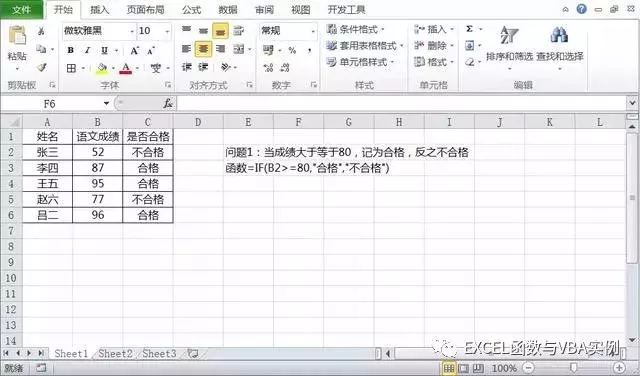=IF(B2>=80,"合格","不合格")

2、多重条件判断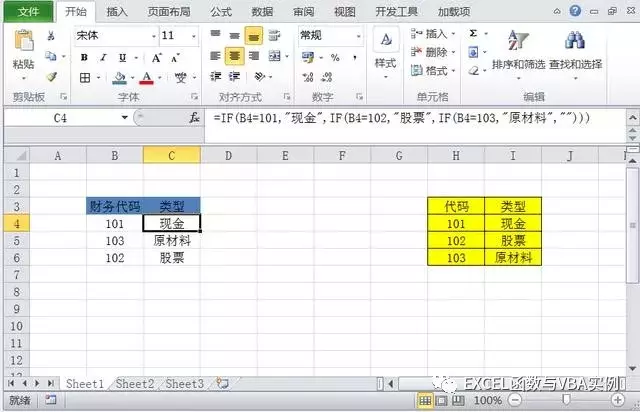=IF(B4=101,"现金",IF(B4=102,"股票",IF(B4=103,"原材料","")))

3、多区间判断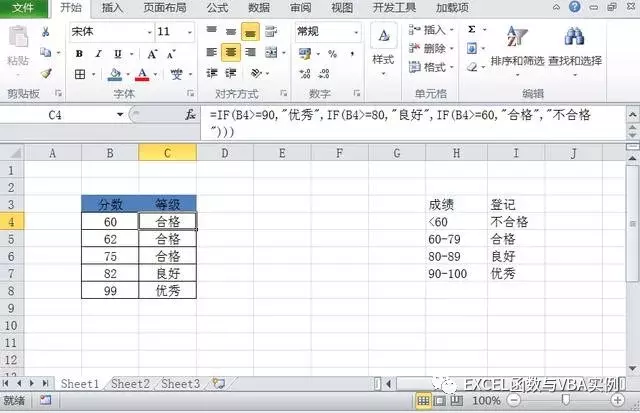=IF(B4>=90,"优秀",IF(B4>=80,"良好",IF(B4>=60,"合格","不合格")))

4、多条件并列判断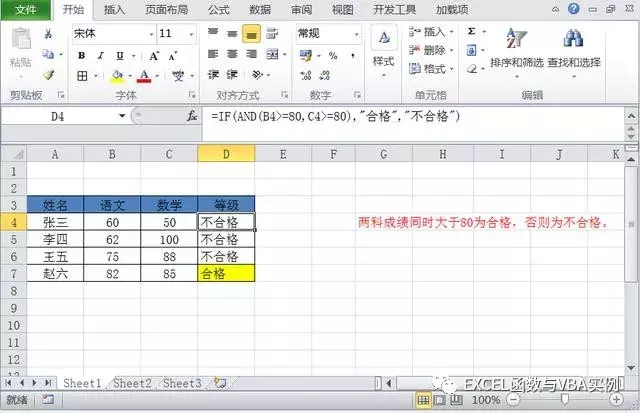IF(AND(B4>=80,C4>=80),"合格","不合格")

5、复杂的多条件判断

=IF(OR(AND(A1>60,B1<100),C1="是"),"合格","不合格")

IF函数结合And和OR进行多条件判断。当多个条件同时成立或者是只要一个条件成立时候如何去操作。

6、判断后返回区域

=VLOOKUP(A1,IF(B1=1,C:D,F:G),2,0)

7、IF({1,0}结构向左查询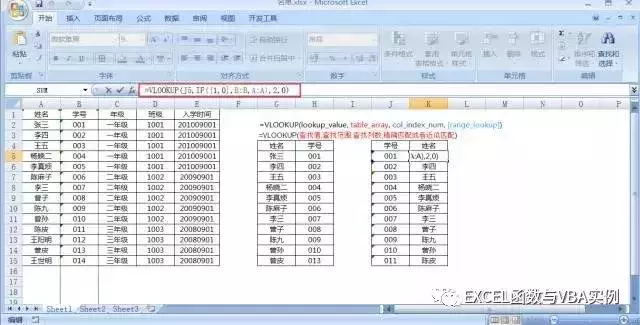=VLOOKUP(J5,IF({1,0},B:B,A:A),2,0)